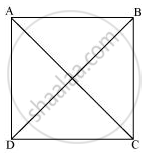Share

# ABCD is a rectangle in which diagonal AC bisects ∠A as well as ∠C. Show that (i) ABCD is a square (ii) diagonal BD bisects ∠B as well as ∠D - Mathematics

Course

#### Question

ABCD is a rectangle in which diagonal AC bisects ∠A as well as ∠C. Show that:-

(i) ABCD is a square (ii) diagonal BD bisects ∠B as well as ∠D.

#### Solution(i) It is given that ABCD is a rectangle.

∴ ∠A = ∠C

⇒ 1/2∠A = 1/2∠C

⇒ ∠DAC = ∠DCA                        (AC bisects ∠A and ∠C)

CD = DA (Sides opposite to equal angles are also equal)

However, DA = BC and AB = CD (Opposite sides of a rectangle are equal)

∴ AB = BC = CD = DA

ABCD is a rectangle and all of its sides are equal.

Hence, ABCD is a square.

(ii) Let us join BD.

In ΔBCD,

BC = CD (Sides of a square are equal to each other)

∠CDB = ∠CBD (Angles opposite to equal sides are equal)

However, ∠CDB = ∠ABD (Alternate interior angles for AB || CD)

∴ ∠CBD = ∠ABD

⇒ BD bisects ∠B.

⇒ ∠CDB = ∠ABD

∴ BD bisects ∠D.

Is there an error in this question or solution?